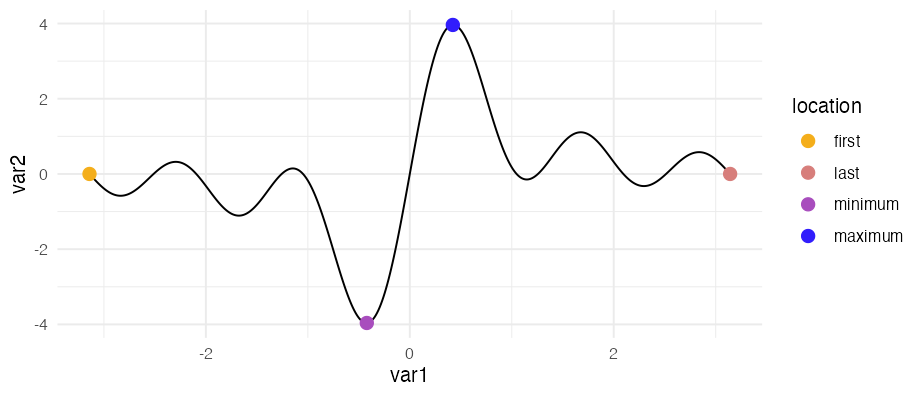ggpointless is an extension of the ggplot2 library providing additional layers. The following functions are implemented in this small package:

• geom_pointless() / stat_pointless(): functions that are making it easy to add minimal emphasis to your plots by means of a point layer.

• geom_lexis() / stat_lexis(): a layer to plot a 45° ‘lifeline’ of an event

## Installation

install.packages("ggpointless")
# Or install the development version
# install.packages("devtools")
devtools::install_github("flrd/ggpointless")

## Using ggpointless

Once you have installed the package, simply attach it by calling:

library(ggpointless)

The main functions in this package are geom_pointless() and geom_lexis(). They work like you are used to from other geom_* functions.

### geom_pointless

Using the functions geom_pointless(), which is a constructor for stat_pointless(), you can highlight the first, or last observations, sample minimum and maximum with the goal to provide some additional context. Or just some visual sugar. geom_pointless() behaves like geom_point() does with the addition of a location argument. You can set it to "first", "last" (default), "minimum", "maximum", and "all", where "all" is just shorthand to select "first", "last", "minimum" and "maximum".

cols <- c('#f4ae1b', '#d77e7b', '#a84dbd', '#311dfc')
x <- seq(-pi, pi, length.out = 500)
y <- outer(x, 1:5, function(x, y) sin(x*y))

df1 <- data.frame(
var1 = x,
var2 = rowSums(y)
)

ggplot(df1, aes(x = var1, y = var2)) +
geom_line() +
geom_pointless(aes(color = after_stat(location)),
location = "all",
size = 3) +
scale_color_manual(values = cols) +
theme_minimal()### geom_lexis

geom_lexis() is a combination of a segment and a point layer. Given a start value and an end value, this geom draws a 45° line which indicates the duration of an event. Required are x and xend aesthetics, y and yend coordinates will be calculated for you.

df2 <- data.frame(
key = c("A", "B", "B", "C", "D"),
x = c(0, 1, 6, 5, 6),
xend = c(5, 4, 10, 8, 10)
)

ggplot(df2, aes(x = x, xend = xend, color = key)) +
geom_lexis(aes(linetype = after_stat(type)), size = .5, point_size = 3) +
coord_equal() +
scale_x_continuous(breaks = c(df2$x, df2$xend)) +
scale_color_manual(values = cols) +
scale_linetype_identity() +
theme_minimal() +
theme(panel.grid.minor = element_blank())See the vignette("ggpointless") for more details.

## Data

The ggpointless package contains the following data sets:

1. co2_ml : CO2 records taken at Mauna Loa
2. covid_vac : COVID-19 Cases and Deaths by Vaccination Status
3. female_leaders : Elected and appointed female heads of state and government

See the vignette("examples") for possible use cases.

## Code of Conduct

Please note that this project is released with a Contributor Code of Conduct. By participating in this project you agree to abide by its terms.